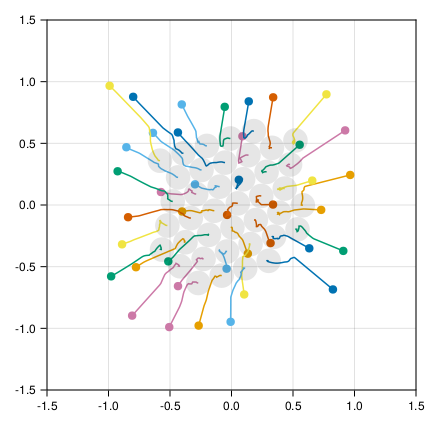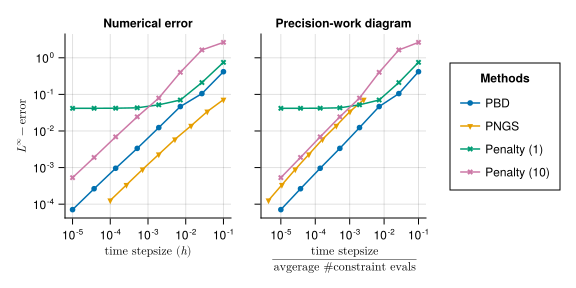# [pre-ANN] DifferentialInclusions.jl

This is a very very early announcement(please let me know if I’m doing bullshit)

I’m working on a package for non-smooth dynamical systems, aka differential inclusions, aka dynamical complementarity problems.

## Current state (almost trivial):

Right now, the package is just a few lines of code and has only one feature:

• It can solve a first-order dynamic complementarity problem of this form
\dot x = f(x) + \sum_{j} \lambda_j \nabla g_j(x) \\ g_j(x) \geq 0, \quad \lambda_j \geq 0, \quad g_j(x) \lambda_j = 0.

with a numerical method that approximates Moreau’s catch-up scheme

x_{k+1} = P_S( x_k + f(x_k) ) \\ \text{where } S = \{ x \in \mathbb{R}^d \mid g_j(x) \geq 0 \quad \text{for all } 1 \leq j \leq m \}.

With a suitable approximation of the projections P_S. That is related to solving linear complementarity problems.

## Next steps:

I’m planning on extending the package to provide:

• first: more solvers (first: classical linear complementarity solvers, then, interfaces to other Julia packages)
• sparse interfaces for constraints (e.g., for non-overlap between objects in contact mechanics)
• later: better, more general interface

## Small demo:

using DifferentialInclusions, OrdinaryDiffEq

cons = (
u -> u,
u -> 2.0 - u,
u -> abs(u - u) - 1.0
)

ode = ODEProblem( (u, p, t) -> -p * u, [0.0, 2.0], (0.0,1.0), [10.0])
prob = DIProblem(ode, cons)

# OSPJ: one-step projected Jacobi method
alg = ProjectiveMethod(OSPJ(),Euler())
sol = solve(prob, alg, dt = 0.001)


## Questions:

If there are related Julia packages, or, if you are interested in such problems, let me knowNote: I’m preparing the package mainly for a publication where I want to compare a few numerical methods.

10 Likes

I commented on the issue. But there is a big push going right now on SciML for complementary problems with @avikpal and a few others in the lab, and it would be good to collaborate on this and get this all fully into the interface.

1 Like

Amazing! I will try to get in touch with @avikpal, and I can discuss more details with him. Of course, I would be very happy to collaborate.

## [Update & Questions]

The package seems to be usable for first-order DI.

• I added the classical projected Gauss-Seidel methods and PSOR.
• I implemented sparse constraints.
• Convergence tests a la DiffEqDevTools.jl is possible (see images for the test case of non-overlapping spheres)

There are a few questions I had during coding (I can also create separate posts if that’s better…):

• Is there a data type for OnceDifferentiable functions which keeps a DiffResult and is type stable? Somehow NLSolversBase.OnceDifferentiable is not type stable with respect to the function f.

• Going further, I’m kind of searching for a way to lazily define ~ N^2 many constraints but with \mathcal{O}(1) memory, e.g., without creating an OnceDifferentiable for each constraint individually. I’m not sure how such an interface could look like… Below is my attempt:

The code below shows my current attempt to implement the constraints

\Vert X_i - X_j \Vert \geq 2R \quad \text{for all } 1 \leq i < j \leq N.

in a way that allows the solver to extract u[:,i] and u[:,j] as static vectors/matrices, such that AD works nice and fast. However, the interface feels a bit complex.

### Example

N = 40
u0 = 2 * ( rand(2, N) .- 0.5 )

cs = let
R = 0.2
f = (u) -> sum(x -> x^2, u[:,1] - u[:,2]) - R^2

con = TSOnceDifferentiable(f, MMatrix{2,2}(zeros(2,2)))
l_inds = LinearIndices(u0)

pairs = ((i,j) for i in 1:N for j in 1:i-1)
pairs_indices = ( SMatrix{2,2,Int64,4}[ [l_inds[:,i] l_inds[:,j]] for (i,j) in pairs  ] )

# one constraint function which will be evaluated for each of the indices
SparseConstraints(pairs_indices,  con)
end

ode = ODEProblem((du,u,p,t) -> (@. du = -p.gamma * u), u0, (0.0,1.0), (gamma = 1.0,))
prob = DIProblem(ode, cs)
alg = ProjectiveMethod(PBD(), Euler())

sol_ = solve(ode, Euler(), dt = 1e-4);  #  3.369 ms (40044 allocations: 22.54 MiB)
sol = solve(prob, alg, dt = 1e-4);      # 85.476 ms (514091 allocations: 30.74 MiB)


Note that solving prob::DIProblem is of course slower than the ode::ODEProblem since one needs to compute ~ N^2 constraints.

### Plots4 Likes